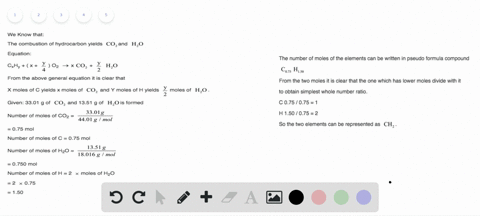### Combustion analysis of a hydrocarbon produced 33.…

01:49University of New Mexico
Problem 106

The molar mass and empirical formula of several compounds are listed. Find the molecular formula of each compound.
a. C4H9, 114.22 g>mol
b. CCl, 284.77 g>mol
c. C3H2N, 312.29 g>mol

a. $\mathrm{C}_{8} \mathrm{H}_{18}$
b. $\mathrm{C}_{6} \mathrm{Cl}_{6}$
c. $\mathrm{C}_{18} \mathrm{H}_{12} \mathrm{N}_{6}$

## Discussion

You must be signed in to discuss.

## Video Transcript

this question is exactly same as the previous potion where we are given with empirical formula. See for EJ night. We can calculate the molar mass carbon corresponds to 12 and hydrogen corresponds to nine on her. Erosion corresponds to one the total Mullah Omar's turns out Toby 114.2 grams. We can determine the molecular formula, which is equal to empirical formula in tow. And we're n stands for number off compounds. We can rearrange this formula in tow. The given form. Let's market as number one, substituting in, substituting in the values into the empirical formula. Toe to toe mine end that is number of compounds, it turns out, an equals two. Hence the monocle formalize multiplied with to giving us C A F 18. Same is to be done for partner will be them. Vehicle formula off Cecile is 284.77 The value off end turns out to be six. We multiply CCL with six, giving us the monocle farm. Last see six see in six for party. The empirical formula is C three edge to and where the more legal Thomas is. 312.29 grams. The end that is number of compound in this case turns out to be six, multiplying the more legal form Love with six giving US C 18 vegetable and six.